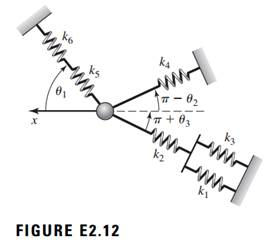### Create an Account

Home / Questions / For the system of translation springs shown in Figure E212 determine the equivalent spring...

# For the system of translation springs shown in Figure E212 determine the equivalent spring constant for motion in the horizontal x direction

For the system of translation springs shown in Figure E2.12, determine the equivalent spring constant for motion in the horizontal (x) direction only. Assume that |∆x| < 1="" so="" that="" the="">θj remain constant.May 16 2020 View more View LessSubscribe To Get Solution